Courses

# NCERT Solutions(Part- 2)- Practical Geometry Class 8 Notes | EduRev

## Class 8 : NCERT Solutions(Part- 2)- Practical Geometry Class 8 Notes | EduRev

The document NCERT Solutions(Part- 2)- Practical Geometry Class 8 Notes | EduRev is a part of the Class 8 Course Class 8 Mathematics by VP Classes.
All you need of Class 8 at this link: Class 8

Question 1. Can you construct the following quadrilateral MIST if we have 100° at M instead of 75°?

Solution: Yes, the quadrilateral MIST can be constructed with M = 100° instead of 75°.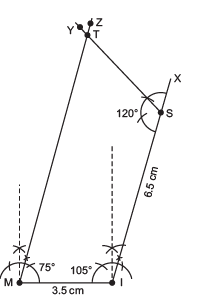Question 2. Can you construct the quadrilateral PLAN if PL = 6 cm, LA = 9.5 cm, P = 75°, L = 150° and A = 140°?

(Hint: Recall angle-sum property)

Solution: Here, P + L + A + N = 75° + 150° + 140° + N
= 365° +
N

But the sum of all the angles of a quadrilateral is 360°.

∴ Construction of quadrilateral PLAN is not possible.

Question 3. In a parallelogram, the lengths of adjacent sides are known. Do we still need measures of the angles to construct as in the Q-1 above?
Solution: No, the measures of three angles are not necessary in case of a parallelogram as its opposite sides are parallel.

EXERCISE 4.1
(Question 1.

MO = 6 cm
OR = 4.5 cm

M = 60°
O = 105°
R = 105°
PL = 4 cm
LA = 6.5 cm
P = 90°
A = 110°
N = 85°
(iii) Parallelogram HEAR
HE = 5 cm
EA = 6 cm

R = 85°
(iv) Rectangle OKAY
OK = 7 cm
KA = 5 cm
Solution: (i) Steps of construction:
I . Draw a line segment MO = 6 cm.
II. At M, draw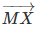, such that OMX = 60°.
III. At O, draw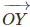, such that MOY = 105°.
IV. From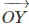, cut off OR = 4.5 cm.
V. At R, draw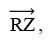, such that ORZ = 105°.
Letintersects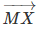at E.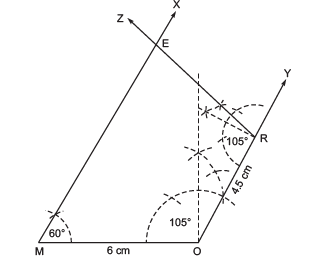Thus, MORE is the required quadrilateral.

(ii) Steps of construction:
I. Draw a line segment AL = 6.5 cm.
II. At A, draw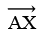such that LAX = 110°.
III. At L, draw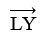such that ALY = 75°.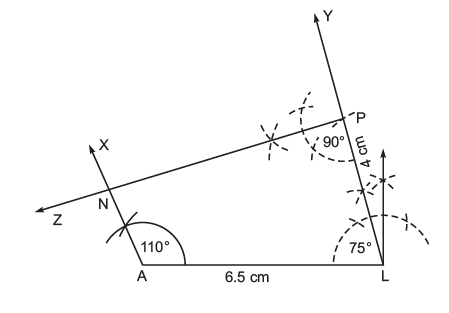Note: L = 75° is not given, but we can determine it using angle sum property
∵ Sum of the three given angles = 110° + 90° + 85° = 285°
∴ The fourth angle L = 360° – 285° = 75°.
IV. From, cut-off LP = 4 cm.
V. At P, draw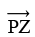such that LPZ = 90°
Let PZ andintersect at N.
Thus, PLAN is the required quadrilateral.
(iii) Steps of construction: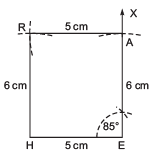I. Draw a line segment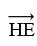= 5 cm.
II. At E, draw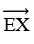such that HEA = 85°.
III. From, cut-off EA = 6 cm.
IV. With centre at A and radius = 5 cm, draw an arc towards H.
V. With centre at H and radius = 6 cm, draw an arc such that it intersects the previous arc at R.
VI. Join RA and RH.
Thus, HEAR is the required quadrilateral.

(iv) Steps of constructions: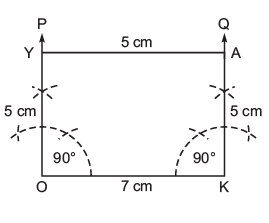I. Draw a line segment OK = 7 cm.
II. At O, draw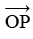such that KOP = 90°.
III. From, cut-off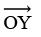= 5 cm.

IV. At K, draw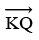such that OKQ = 90°.

V. Fromcut-off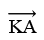= 5 cm.
VI. Join A and Y.
Thus, OKAY is the required quadrilateral.

CONSTRUCTION OF A QUADRILATERAL WHEN THREE SIDES AND TWO INCLUDED ANGLES ARE GIVEN

Question. We used some five measurements to draw quadrilateral so far. Can there be different sets of five measurements (other than seen so far) to draw a quadrilateral? The following problems may help you in answering the question.
(i) Quadrilateral ABCD with AB = 5 cm, BC = 5.5 cm, CD = 4 cm, AD = 6 cm and ∠B = 80°.
(ii) Quadrilateral PQRS with PQ = 4.5 cm, ∠P = 70°,∠Q = 100°, ∠ R = 80° and ∠S = 110°.
Construct a few more examples of your own to find sufficiency/insufficiency of the data for construction of a quadrilateral
Solution: (i) It is possible to construct a quadrilateral ABCD with the given measurements.
(ii) Not possible, because we cannot locate the points R and S with the help of given measurements.

EXERCISE 4.4
Question 1 Construct the following quadrilaterals.
DE = 4 cm
EA = 5 cm
AR = 4.5 cm
∠E = 60°
∠A = 90°
TR = 3.5 cm
RU = 3 cm
UE = 4 cm
∠R = 75°
∠U = 120°
Solution: (i) Steps of construction:

I. Draw a line segment DE = 4 cm.
II. At E, draw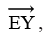such that ∠DEX = 60°.
III. From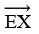, cut-off  EA = 5 cm.
IV. At A, draw ray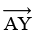such that ∠EAY = 90°.
V. From, cut-off AR = 4.5 cm.
VI. Join R and D.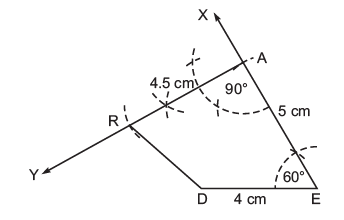Thus, DEAR is the required quadrilateral.

(ii) Steps of construction:

I. Draw a line segment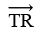= 3.5 cm.

II. At R, draw a ray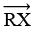such that ∠TRX = 75°.
III. From, cut-off RU = 3 cm.
IV. At U, draw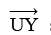such that ∠RUY = 120°.
V. From UY, cut-off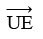= 4 cm.
VI. Join E and T.
Thus, TRUE is the required quadrilateral.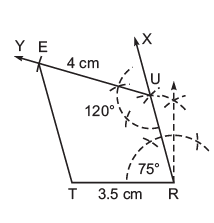SOME SPECIAL CASES

Using the properties of a square, rectangle, kite and rhombus, etc., we can construct some special quadrilaterals even with less than 5 measurements.

Offer running on EduRev: Apply code STAYHOME200 to get INR 200 off on our premium plan EduRev Infinity!

89 docs|16 tests

,

,

,

,

,

,

,

,

,

,

,

,

,

,

,

,

,

,

,

,

,

;